# Bihar Board Class 10th Maths Solutions Chapter 7 Coordinate Geometry Ex 7.2

Bihar Board Class 10th Maths Solutions Chapter 7 Coordinate Geometry Ex 7.2 Textbook Questions and Answers.

## BSEB Bihar Board Class 10th Maths Solutions Chapter 7 Coordinate Geometry Ex 7.2Question 1.
Find the coordinates of the point which divides the join of (- 1, 7) and (4, – 3) in the ratio 2:3.
Solution:
Let P(x, y) be the required point.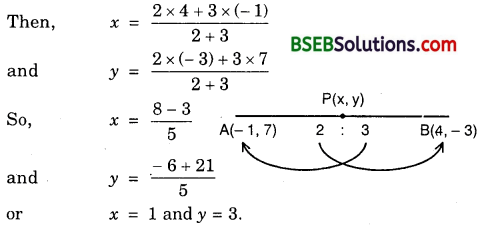So, the coordinates of P are (1, 3).

Question 2.
Find the coordinates of the points of trisection of the lire segment joining (4, – 1) and (- 2, – 3).
Solution:
Let P and Q be the points of trisection of the line segment joining A(4, – 1) and B(- 2, – 3). Then, AP = PQ = QB = k (say).
∴ PB = PQ + QB = 2k
and AQ = AP + PQ = 2k
So, AP : PB = k : 2k = 1 : 2 and AQ : QB = 2k : k = 2 : 1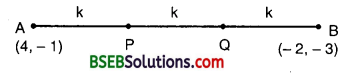So, P divides AB internally in the ratio 1 : 2, while Q divides AB internally in the ratio 2 : 1. Thus, the coordinates of P are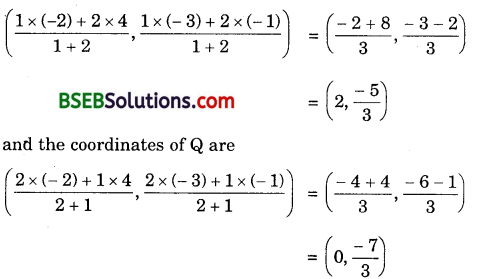Hence, the two points of trisection are
(2, $$\frac { -5 }{ 3 }$$) and (0, $$\frac { -7 }{ 3 }$$).Question 3.
To conduct Sports Day activities in your rectangular shaped school ground ABCD, lines have been drawn with chalk powder at a distance of 1 m each. 100 flower pots have been placed at a distance of 1 m from each other along AD, as shown in figure.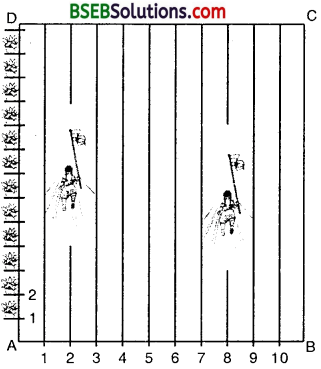Niharika runs $$\frac { 1 }{ 4 }$$ th the distance AD on the 2nd line and posts a green flag. Preet runs $$\frac { 1 }{ 5 }$$ th the distance AD on the eighth line and posts a red flag. What is the distance between both the flags ? If Rashmi has to post a blue flag exactly halfway between the line (segment) joining the two flags, where should she post her flag ?
Solution:
Clearly from the figure, the position of green flag posted by Niharika is given by P(2, $$\frac { 1 }{ 4 }$$ x 100) i.e., P(2, 25) and that of red, flag posted by Preet is given by Q(8, $$\frac { 1 }{ 5 }$$ x 100), i.e., Q(8, 20)
Now, PQ = $$\sqrt{(8-2)^{2}+(20-25)^{2}}$$ = $$\sqrt{(6)^{2}+(-5)^{2}}$$
= $$\sqrt{36+25}$$
= $$\sqrt{61}$$
∴ The distance between the flags = $$\sqrt{61}$$ metres
Let M be the position of the blue flag posted by Rashmi in the halfway of line segment PQ.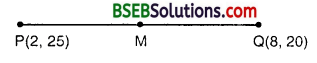∴ M is the given by $$\left(\frac{2+8}{2}, \frac{25+20}{2}\right)$$
or $$\left(\frac{10}{2}, \frac{45}{2}\right)$$, i.e., (5, 22.5)
Thus, the blue flag should be on the fifth line at a distance of 22.5 m above it.

Question 4.
Find the ratio in which the line segment joining the points (- 3, 10) and (6, – 8) is divided by (- 1, 6).
Solution:
Let the point P(- 1, 6) divide the line joining A(- 3, 10) and B(6, – 8) in- the ratio k : 1. Then, the coordinates of P are $$\left(\frac{6 k-3}{k+1}, \frac{-8 k+10}{k+1}\right)$$
But, the coordinates of P are given as (- 1, 6).Hence, the point P divides AB in the ratio 2 : 7.Question 5.
Find the ratio in which the line segment joining A(1, – 5) and B(- 4, 5) is divided by the x-axis. Also, find the coordinates of the point of division.
Solution:
Let the required ratio be k : 1. Then, the coordinates of the point P of division are $$\left(\frac{-4 k+1}{k+1}, \frac{5 k-5}{k+1}\right)$$But it is a point on x-axis on which y-coordinate of every point is 0.
∴ $$\frac{5 k-5}{k+1}$$ = 0
or 5k – 5 = 0
5k = 5
k = 1
Thus, the required ratio is 1 : 1 and the point of division P is given by
$$\left(\frac{-4 \times 1+1}{1+1}, 0\right)$$, i.e., $$\left(\frac{-4+1}{2}, 0\right)$$ i.e., $$\left(\frac{-3}{2}, 0\right)$$

Question 6.
If (1, 2), (4, y), (x, 6) and (3, 5) are the vertices of a parallelogram taken in order, find x and y.
Solution:
Let A(1, 2), B(4, y), C(x, 6) and D(3, 5) be the vertices of a parallelogram ABCD.
Since the diagonals of a parallelogram bisect each other, therefore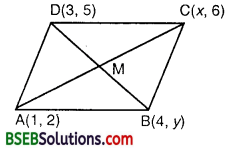$$\frac{x+1}{2}$$ = $$\frac{3+4}{2}$$
or x + 1 = 7 or x = 6
and $$\frac{5+y}{2}$$ = $$\frac{6+2}{2}$$
or 5 + y = 8
or y = 3
Hence, x = 6 and y = 3.

Question 7.
Find the coordinates of a point A, where AB is the diameter of a circle whose centre is (2, – 3) and B is (1, 4).
Solution:
Let AB be a diameter of the circle having its centre at C(2, – 3) such that the coordinates of end B are (1, 4).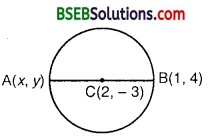Let the coordinates of A be (x, y).
Since C is the mid-point of AB, therefore
the coordinates of C are $$\left(\frac{x+1}{2}, \frac{y+4}{2}\right)$$
But, the coordinates of C are given as (2, – 3).
∴ $$\frac{x+1}{2}$$ = 2 or x + 1 = 4 or x = 3
and $$\frac{y+4}{2}$$ = – 3 or y + 4 = – 6 or y = – 10
Hence, the coordinates of A are (3, – 10).Question 8.
If A and B are (- 2, – 2) and (2, – 4) respectively, find the coordinates of P such that AP = lies on the line segment AB.
Solution:
We have : AP = $$\frac{3}{7}$$ABLet P(x, y) be the point which divides the join of A(- 2, – 2) and B(2, – 4) in the ratio 3:4.Hence, the coordinates of the point P are $$. Question 9. Find the coordinates of the points which divide the line segment joining A(- 2, 2) and B(2, 8) into four equal parts. Solution: Let P1 P2 and P3 be the points that divide the line segment joining A(- 2, 2) and B(2, 8) into four equal parts.Since P1 divides the line segment into two equal parts, therefore coordinates of P2 (i.e., mid-point) are [latex]\left(\frac{-2+2}{2}, \frac{2+8}{2}\right)$$, i.e., (0, 5).
Now, P1 divides the line segment AP2 into two equal parts.
Coordinates of P1 (i.e., mid-point) are $$\left(\frac{-2+0}{2}, \frac{2+5}{2}\right)$$, i,e., $$\left(-1, \frac{7}{2}\right)$$,
Again, P3 is the mid-point of line segment P2B.
∴ Coordinates or P3 are $$\left(\frac{0+2}{2}, \frac{5+8}{2}\right)$$, i.e., (1, $$\frac{13}{2}$$).Question 10.
Find the area of a rhombus if its vertices are (3, 0), (4, 5), (- 1, 4) and (- 2, – 1) taken in order.
Solution:
Let A(3, 0), B(4, 5), C(- 1, 4) and D(- 2, – 1) be the vertices of the rhombus ABCD.